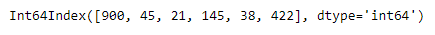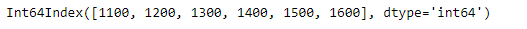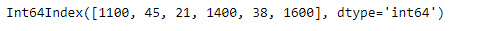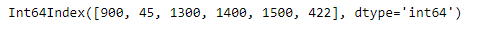GFG App
Open AppBrowser
Continue

# Python | Pandas Index.where

Pandas Index is an immutable ndarray implementing an ordered, sliceable set. It is the basic object which stores the axis labels for all pandas objects.

Pandas` Index.where` function return an Index of same shape as self and whose corresponding entries are from self where cond is True and otherwise are from other.

Syntax: Index.where(cond, other=None)

Parameter :
cond : boolean array-like with the same length as self
other : scalar, or array-like

Returns : Index

Example #1: Use `Index.where` function to return an Index, in which we select the value from the other Index if value of this Index is not smaller than 100.

 `# importing pandas as pd ` `import` `pandas as pd ` ` `  `# Creating the first index ` `idx1 ``=` `pd.Index([``900``, ``45``, ``21``, ``145``, ``38``, ``422``]) ` ` `  `# Creating the second index ` `idx2 ``=` `pd.Index([``1100``, ``1200``, ``1300``, ``1400``, ``1500``, ``1600``]) ` ` `  `# Print the first index ` `print``(idx1) ` ` `  `# Print the second index ` `print``(idx2) `

Output :Now we will use `Index.where` function to return an Index, in which we select the value from the other Index if value of this Index is not smaller than 100.

 `# return the new index based on the condition ` `result ``=` `idx1.where(idx1 < ``100``, idx2) ` ` `  `# Print the result ` `print``(result) `

Output :As we can see in the output, the `Index.where` function has successfully returned an Index object satisfying the passed condition.

Example #2 : Use `Index.where` function to return an Index, which satisfy the passed condition.

 `# importing pandas as pd ` `import` `pandas as pd ` ` `  `# Creating the first index ` `idx1 ``=` `pd.Index([``900``, ``45``, ``21``, ``145``, ``38``, ``422``]) ` ` `  `# Creating the second index ` `idx2 ``=` `pd.Index([``1100``, ``1200``, ``1300``, ``1400``, ``1500``, ``1600``]) ` ` `  `# Print the first index ` `print``(idx1) ` ` `  `# Print the second index ` `print``(idx2) `

Output :Now we will use `Index.where` function to return an Index, in which we select the value from the other Index if value of other Index minus 1200 is not smaller than idx1.

 `# return the new index based on the condition ` `result ``=` `idx1.where((idx2 ``-` `1200``) < idx1, idx2) ` ` `  `# Print the result ` `print``(result) `

Output :As we can see in the output, the `Index.where` function has successfully returned an Index object satisfying the passed condition.

My Personal Notes arrow_drop_up